# How to count the number of stars in a photo?

Hello everyone!

Recently I participated in the Olympiad on artificial intelligence in Python and there were many interesting problems, but the most interesting one is about the stars in the sky: “Given a photo of the starry sky from the ground. Task: determine the number of stars in the sky.”

It seems to be not difficult if the photo is only with stars, for example:

Okay, everything is easy here! It can be solved like this:

Importing libraries

``````from scipy.spatial import distance
from skimage import io
from skimage.feature import blob_dog, blob_log, blob_doh
from skimage.color import rgb2gray

import matplotlib.pyplot as plt``````

I will use the library `skimage` to work with the image, `scipy` – for complex mathematical calculations and `matplotlib.pyplot` for debug output.

``````image = io.imread(input("Путь до изображения: "))
image_gray = rgb2gray(image)``````

Let’s open the image and convert it to black and white for its simplicity and its future processing.

To understand how we simplified the image representation, let’s take the first pixel in RGB and GrayScale:

``````print(image[0, 0])
print(image_gray[0, 0])``````

And we get:

``````[24 16 14] #RGB
0.06884627450980392 #GrayScale``````

floats are easier to work with than tuples

Next, we need to figure out how to look for stars. Fortunately, in the module `skimage` there is a function for detecting blobs. There are three types of them:

• Laplacian of Gaussian (LoG)

• Difference of Gaussian (DoG)

• Determinant of Hessian (DoH)

From personal experience and comparing the results, I came to the conclusion that for this task I will use with such parameters.

``blobs_log = blob_log(image_gray, max_sigma=20, num_sigma=10, threshold=.05)``

Next, I mark the points in the picture and count their number.

``````fig = plt.figure()

ax.set_title('Laplacian of Gaussian')
ax.imshow(image)
c_stars = 0
for blob in blobs_log:
y, x, r = blob
if r > 2:
continue
ax.add_patch(plt.Circle((x, y), r, color="purple", linewidth=2, fill=False))
c_stars += 1
print("Количество звёзд: " + str(c_stars))
ax.set_axis_off()
plt.tight_layout()
plt.show()``````

Running, I get this result:

``Количество звёзд: 353``

But will the program work correctly if you enter a picture for it that corresponds to the condition of the problem?

And we will get a lot of false points.

## Algorithm improvement

Therefore, it is necessary to improve the point search algorithm. To do this, we will use one more feature of the library `skimage` it is image segmentation.

Here is a link to a source, which describes the basics of image segmentation.

Taking the necessary piece of code from there, we improve the current algorithm.

We import new modules:

``````from skimage.segmentation import slic, mark_boundaries
import numpy as np
from sklearn.cluster import KMeans``````

We segment the image using the function `slic`

``````segments = slic(img, start_label=0, n_segments=200, compactness=20)
segments_ids = np.unique(segments)
print(segments_ids)

# centers
centers = np.array([np.mean(np.nonzero(segments == i), axis=1) for i in segments_ids])
print(centers)
vs_right = np.vstack([segments[:, :-1].ravel(), segments[:, 1:].ravel()])
vs_below = np.vstack([segments[:-1, :].ravel(), segments[1:, :].ravel()])
bneighbors = np.unique(np.hstack([vs_right, vs_below]), axis=1)

fig = plt.figure(figsize=(10, 10))
plt.imshow(mark_boundaries(img, segments))
plt.scatter(centers[:, 1], centers[:, 0], c="y")

for i in range(bneighbors.shape):
y0, x0 = centers[bneighbors[0, i]]
y1, x1 = centers[bneighbors[1, i]]

l = Line2D([x0, x1], [y0, y1], alpha=0.5)
``````

Create a dictionary to determine which segment each pixel belongs to.

``````dict_seg = {}
for i in range(img.shape):
for j in range(img.shape):
seg = segments[i, j]
if seg not in dict_seg.keys():
dict_seg[seg] = [img[i, j]]
continue
dict_seg[seg].append(img[i, j])``````

We calculate the average color for each segment

``````def middle(a, b):
color = []
for i, j in zip(a, b):
color.append((i + j) // 2)
return color

for k, v in dict_seg.items():
# вычисляем перцентиль для выброса пересвеченных пикселей в сегменте
p = int(0.9 * len(v))
v = sorted(list(v), key=lambda x: my_distance(x, white))
s = [0, 0, 0]
for c in v:
s += c
s += c
s += c
s //= len(v[:p])
s //= len(v[:p])
s //= len(v[:p])
dict_seg[k] = s``````

At the output, we get a dictionary with average colors in each segment.

``>>> {0: [5, 3, 14], 1: [5, 3, 16], 2: [7, 4, 17] ... 190: [23, 19, 37]}``

Next, we cluster the dictionary `dict_seg`by using `KMeans` from the library `sklearn`

``````kmeans = KMeans(n_clusters=3, algorithm="elkan")
kmeans.fit(list(dict_seg.values()))
labels, counts = np.unique(kmeans.labels_, return_counts=True)``````

Create a new dictionary of the form `{segment: claster_num(их всего 3)}`

``````dic_seg_claster = {}
for key, value in dict_seg.items():
dic_seg_claster[key] = kmeans.predict(Hello everyone!Recently I participated in the Olympiad on artificial intelligence in Python and there were many interesting problems, but the most interesting one is about the stars in the sky: "Given a photo of the starry sky from the ground. Task: determine the number of stars in the sky."  It seems to be not difficult if the photo is only with stars, for example: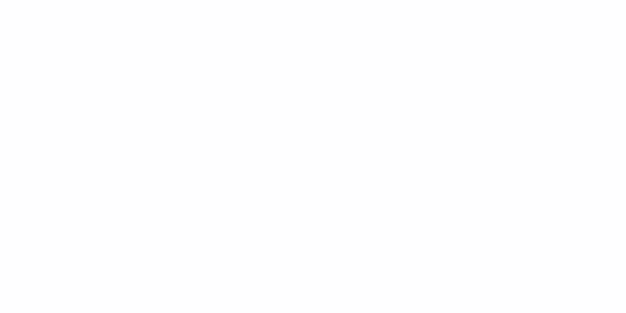Photos with only starsOkay, everything is easy here!  It can be solved like this:Importing librariesfrom scipy.spatial import distance
from skimage import io
from skimage.feature import blob_dog, blob_log, blob_doh
from skimage.color import rgb2gray

import matplotlib.pyplot as pltI will use the library skimage to work with the image, scipy - for complex mathematical calculations and matplotlib.pyplot  for debug output.image = io.imread(input("Путь до изображения: "))
image_gray = rgb2gray(image)Let's open the image and convert it to black and white for its simplicity and its future processing.To understand how we simplified the image representation, let's take the first pixel in RGB and GrayScale:print(image[0, 0])
print(image_gray[0, 0])And we get:[24 16 14] #RGB
0.06884627450980392 #GrayScalefloats are easier to work with than tuples  Next, we need to figure out how to look for stars.  Fortunately, in the module skimage  there is a function for detecting blobs.  There are three types of them:  Laplacian of Gaussian (LoG)Difference of Gaussian (DoG)Determinant of Hessian (DoH)You can read more about their differences. here...From personal experience and comparing the results, I came to the conclusion that for this task I will use with such parameters.blobs_log = blob_log(image_gray, max_sigma=20, num_sigma=10, threshold=.05)Next, I mark the points in the picture and count their number.fig = plt.figure()

ax.set_title('Laplacian of Gaussian')
ax.imshow(image)
c_stars = 0
for blob in blobs_log:
y, x, r = blob
if r > 2:
continue
ax.add_patch(plt.Circle((x, y), r, color="purple", linewidth=2, fill=False))
c_stars += 1
print("Количество звёзд: " + str(c_stars))
ax.set_axis_off()
plt.tight_layout()
plt.show()Running, I get this result:Количество звёзд: 353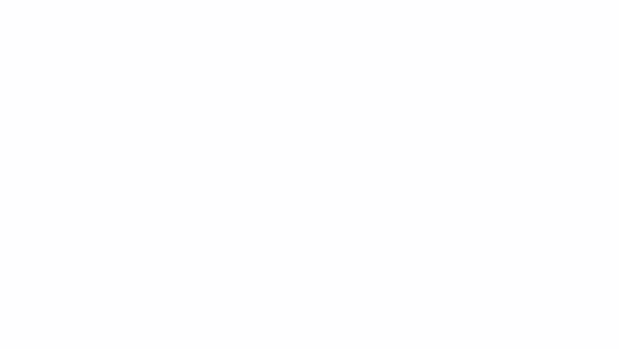Program outputBut will the program work correctly if you enter a picture for it that corresponds to the condition of the problem?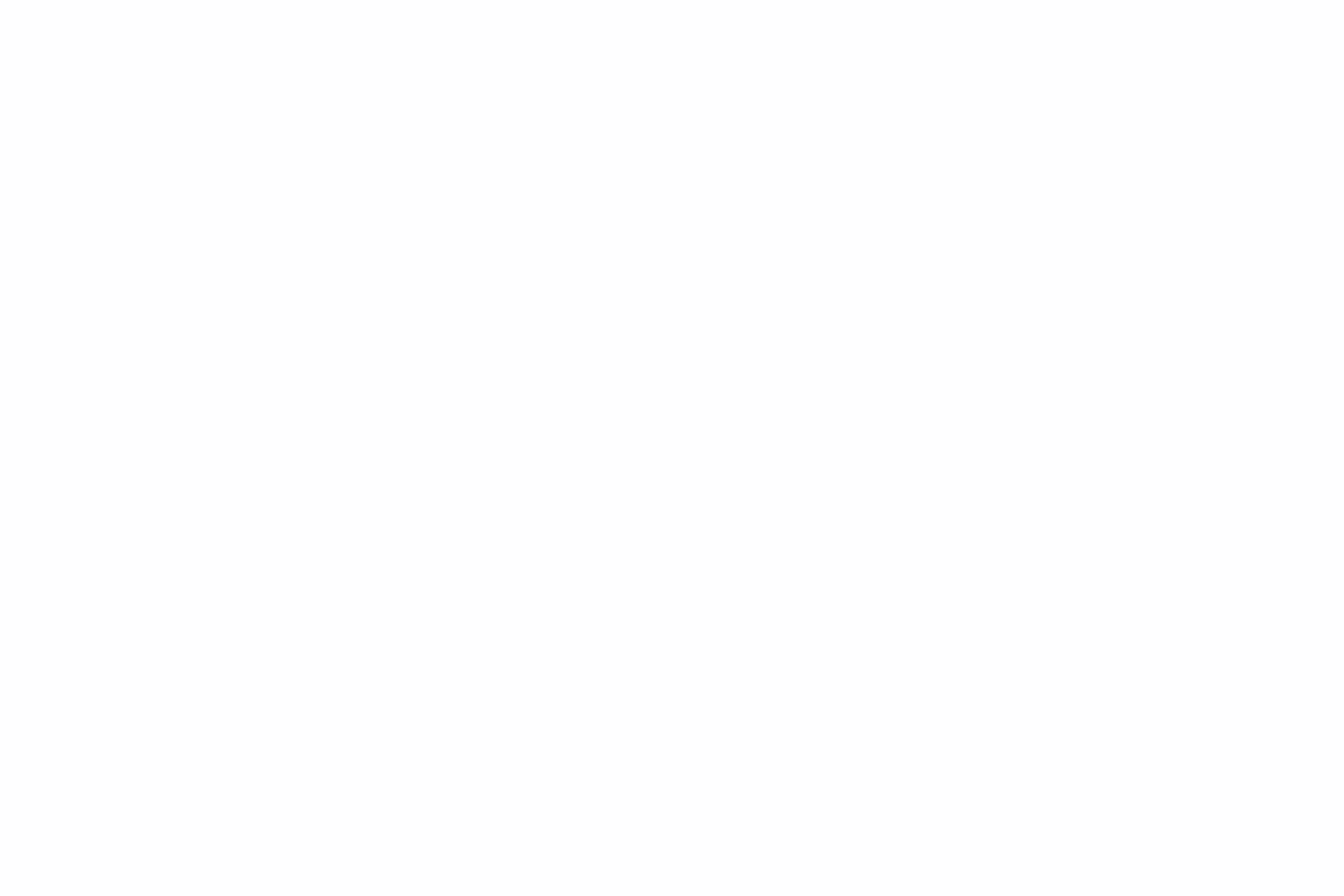Picture corresponding to the taskAnd we will get a lot of false points.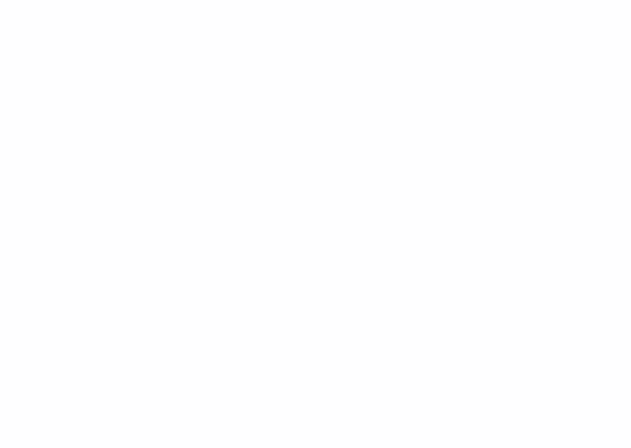Algorithm improvementTherefore, it is necessary to improve the point search algorithm.  To do this, we will use one more feature of the library skimage  it is image segmentation.Here is a link to a source, which describes the basics of image segmentation.Taking the necessary piece of code from there, we improve the current algorithm.We import new modules:from skimage.segmentation import slic, mark_boundaries
import numpy as np
from sklearn.cluster import KMeansWe segment the image using the function slicsegments = slic(img, start_label=0, n_segments=200, compactness=20)
segments_ids = np.unique(segments)
print(segments_ids)

# centers
centers = np.array([np.mean(np.nonzero(segments == i), axis=1) for i in segments_ids])
print(centers)
vs_right = np.vstack([segments[:, :-1].ravel(), segments[:, 1:].ravel()])
vs_below = np.vstack([segments[:-1, :].ravel(), segments[1:, :].ravel()])
bneighbors = np.unique(np.hstack([vs_right, vs_below]), axis=1)

fig = plt.figure(figsize=(10, 10))
plt.imshow(mark_boundaries(img, segments))
plt.scatter(centers[:, 1], centers[:, 0], c="y")

for i in range(bneighbors.shape):
y0, x0 = centers[bneighbors[0, i]]
y1, x1 = centers[bneighbors[1, i]]

l = Line2D([x0, x1], [y0, y1], alpha=0.5)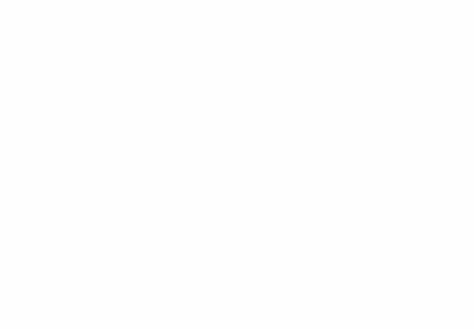Image segmentationCreate a dictionary to determine which segment each pixel belongs to.dict_seg = {}
for i in range(img.shape):
for j in range(img.shape):
seg = segments[i, j]
if seg not in dict_seg.keys():
dict_seg[seg] = [img[i, j]]
continue
dict_seg[seg].append(img[i, j])We calculate the average color for each segmentdef middle(a, b):
color = []
for i, j in zip(a, b):
color.append((i + j) // 2)
return color

for k, v in dict_seg.items():
# вычисляем перцентиль для выброса пересвеченных пикселей в сегменте
p = int(0.9 * len(v))
v = sorted(list(v), key=lambda x: my_distance(x, white))
s = [0, 0, 0]
for c in v:
s += c
s += c
s += c
s //= len(v[:p])
s //= len(v[:p])
s //= len(v[:p])
dict_seg[k] = sAt the output, we get a dictionary with average colors in each segment.>>> {0: [5, 3, 14], 1: [5, 3, 16], 2: [7, 4, 17] ... 190: [23, 19, 37]}Next, we cluster the dictionary dict_segby using KMeans from the library sklearnkmeans = KMeans(n_clusters=3, algorithm="elkan")
kmeans.fit(list(dict_seg.values()))
labels, counts = np.unique(kmeans.labels_, return_counts=True)Create a new dictionary of the form {segment: claster_num(их всего 3)}dic_seg_claster = {}
for key, value in dict_seg.items():
dic_seg_claster[key] = kmeans.predict([value])
max_l = max(dic_seg_claster.values(), key=lambda x: list(dic_seg_claster.values()).count(x))Find the most frequent cluster in the pictureNext comes our previous code, but with some changes:blobs_log = blob_log(image_gray, max_sigma=30, num_sigma=10, threshold=.05)
fig = plt.figure()
...
for blob in blobs_log:
y, x, r = blob
# новый фрагмент
if dic_seg_claster[segments[int(y), int(x)]] == max_l:
c = plt.Circle((x, y), r, color="purple", linewidth=2, fill=False)
count += 1
...And we are already getting a better result.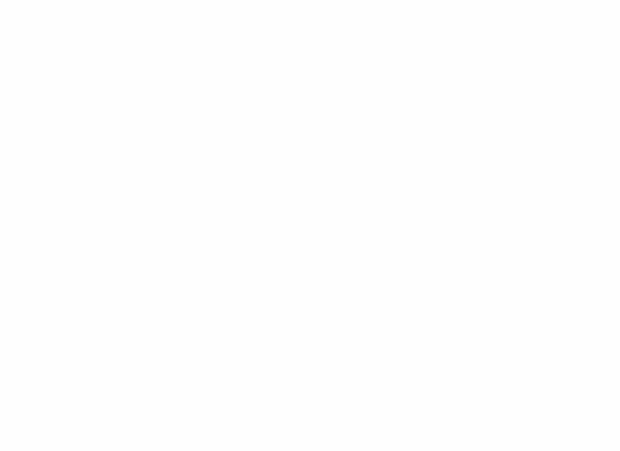1418 starsAfter calculating the statistical probability, I came to the conclusion that the errors on unnecessary objects are compensated for by unselected stars.This algorithm can still be improved for a long time, adjusting the number of segments and clusters.  But for the moment I will pause.Leave all your wishes or indignations in the comments, it will be very interesting for me to read them in order to improve my algorithm to an ideal state)The finished project can be found in gitHubThank you for the attention!``````)
max_l = max(dic_seg_claster.values(), key=lambda x: list(dic_seg_claster.values()).count(x))```Find the most frequent cluster in the pictureNext comes our previous code, but with some changes:```blobs_log = blob_log(image_gray, max_sigma=30, num_sigma=10, threshold=.05)
fig = plt.figure()